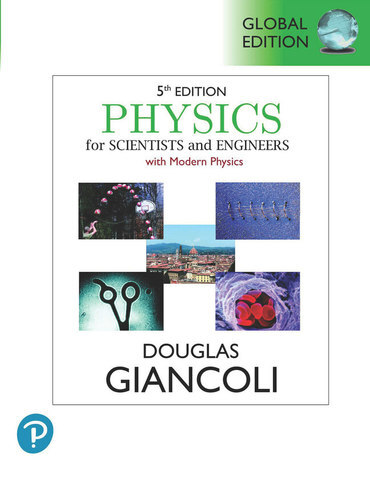# Physics for Scientists & Engineers with Modern Physics Douglas Giancoli 5th GE 9781292440279

RM 225.00

TITLE :Physics for Scientists & Engineers with Modern Physics - Giancoli

ISBN13 :9781292440279

PUBLISHER : PEARSON (2023)

EDITION : 5TH GE PAPERBACK

PAGES : 1465 COLOR PAGES

1. Introduction, Measurement, Estimating
2. Describing Motion: Kinematics in One Dimension
3. Kinematics in Two or Three Dimensions; Vectors
4. Dynamics: Newton's Laws of Motion
5. Using Newton's Laws: Friction, Circular Motion, Drag Forces
6. Gravitation and Newton's Synthesis
7. Work and Energy
8. Conservation of Energy
9. Linear Momentum
10. Rotational Motion
11. Angular Momentum; General Rotation
12. Static Equilibrium; Elasticity and Fracture
13. Fluids
14. Oscillations
15. Wave Motion
16. Sound
17. Temperature, Thermal Expansion, and the Ideal Gas Law
18. Kinetic Theory of Gases
19. Heat and the First Law of Thermodynamics
20. Second Law of Thermodynamics
21. Electric Charge and Electric Field
22. Gauss's Law
23. Electric Potential
24. Capacitance, Dielectrics, Electric Energy Storage
25. Electric Current and Resistance
26. DC Circuits
27. Magnetism
28. Sources of Magnetic Field
29. Electromagnetic Induction and Faraday's Law
30. Inductance, Electromagnetic Oscillations, and AC Circuits
31. Maxwell's Equations and Electromagnetic Waves
32. Light: Reflection and Refraction
33. Lenses and Optical Instruments
34. The Wave Nature of Light: Interference and Polarization
35. Diffraction
36. The Special Theory of Relativity
37. Early Quantum Theory and Models of the Atom
38. Quantum Mechanics
39. Quantum Mechanics of Atoms
40. Molecules and Solids